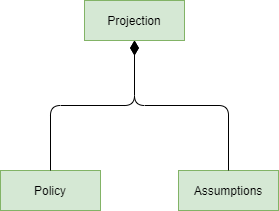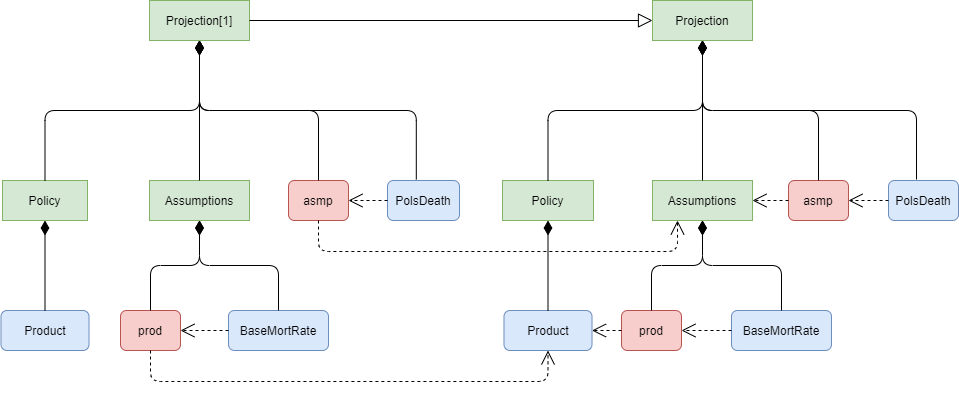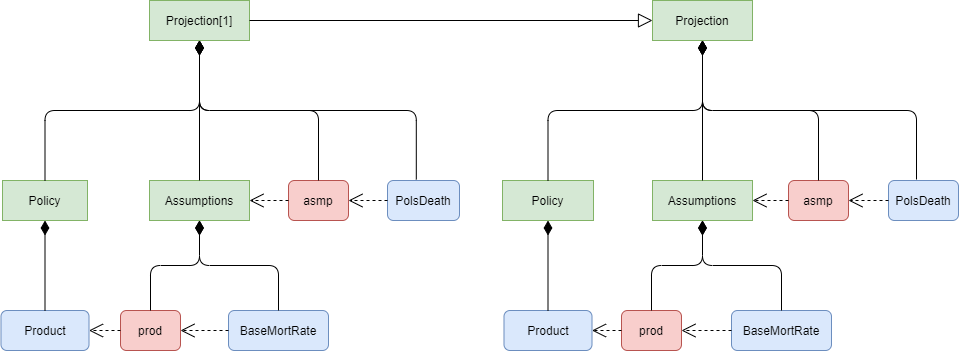# modelx v0.10.0 will make lifelib simpler

Sep 19, 2020 • Fumito Hamamura

modelx v0.10.0 introduces relative reference, a new way of referencing modelx objects in setting values of derived References. This post explains how relative References can help simplify modelx models.

The code below shows the formula property of the `Projection` space in the simplelife model from lifelib v0.14.0.

``````>>> simplelife.Projection.formula
def _formula(PolicyID, ScenID=1):
refs = {'pol': Policy[PolicyID],
'asmp': Assumption[PolicyID],
'scen': Economic[ScenID],
'DiscRate': Economic[ScenID].DiscRate}
return {'refs': refs}
``````

The formula has always been that convoluted since the first release of lifelib and I wanted to make it simpler and easier to understand. I had thought about how to enhance modelx so that the formula above could be stripped down to a simpler expression. After a long while I finally came up with the idea of relative reference and implemented in modelx v0.10.0, which was released a couple days ago. The concept of relative and absolute reference is somewhat similar to that of spreadsheets.

With the introduction of relative reference, the formula can be as simple as below.

``````>>> newlife.Projection.formula
lambda PolicyID, ScenID=1: None
``````

## How relative reference works

Let’s say you want to build a simple cashflow projection model of life insurance policies, one that’s similar to but much simpler than simplelife.

You would create a Space to put Cells for projection calculations in it. Let’s name the Space `Projection` here. You could put all the Cells needed for projection in `Projection`, but to avoid filling the Space with too many Cells, you would decide to create 2 child spaces `Policy` and `Assumptions`, and put Cells representing policy attributes in `Policy`, and put Cells representing assumptions in `Assumptions`.For example, Projection would contain `PolsDeath`, Number of Death, which need to refer to `BaseMortRate` in its formula. `BaseMortRate` is in `Assumptions`, and in turn it needs to refer to `Product`, Product ID of the selected policy, which is in `Policy`.

`PolsDeath` could refer to `BaseMortRate` in its formula as `Assumptions.BaseMortRate`, but it’s too verbose, so you decide to define an alias for `Assumptions` as a Reference, and name it `asmp`, then you get to refer to `BaseMortRate` in `PolsDeath`’s formula as `asmp.BaseMortRate`.

In order for `BaseMortRate` to be able to refer to `Product` in `Policy`, `Product` needs to be visible from `Assumptions`. One way to achieve this is to define a Reference that is bound to `Product`. Let’s name it `prod` here.Then you want to parameterize `Projection` by `PolicyID`, so that the projection for each model point can be expressed as `Projection[PolicyID]`, such as `Projection`, `Projection`, etc..

You would think that setting the parameter as below would work:

``````>>> Projection.parameters = ("PolicyID",)

>>> Projection.formula
lambda PolicyID: None
``````

But this does not work with modelx v0.9.0 or older, because in `Projection`, derived References, such as `asmp` and `prod` reference the same objects as what `asmp` and `prod` in `Projection` reference, which are in the base Space, `Projection`.With modelx v0.10.0, this behavior is changed.

When References are set by the assignment operation, the References are set in auto mode. In this example, `asmp` and `prod` are auto References. When `Projection` explicitly inherits from `Projection`, `asmp` and `prod` in `Projection` are derived from `asmp` and `prod` in `Projection`. Since `Projeciton.asmp` and `Projection.prod` are auto References, their derived References, `Projection.asmp` and `Projection.prod` are bound to objects in `Projections`, whose relative names in `Projection` are consistent with the relative names of the values of the base References in `Projection`.

If `Projeciton.asmp` or `Projction.prod` were bound to Spaces or Cells outside `Projection`, then `Projection.asmp` and `Projection.prod` would reference the same Spaces or Cells as the values of the base References. If `asmp` and `prod` were set in relative mode, then creating `Projection` would raise an Error, because relative reference would be impossible.## lifelib will become simpler

With the introduction of relative reference, lifelib models will be simpler. I haven’t updated the lifelib package yet, but I developed an experimental implementation of an updated simplelife as newlife. A refined version of the newlife will replace the current simplelife.

In the current version (0.0.14) of the simplelife model, `Projection` space has the following formula as I mentioned earlier.

``````>>> simplelife.Projection.formula
def _formula(PolicyID, ScenID=1):
refs = {'pol': Policy[PolicyID],
'asmp': Assumption[PolicyID],
'scen': Economic[ScenID],
'DiscRate': Economic[ScenID].DiscRate}
return {'refs': refs}
``````

The `Policy` and `Assumption` Spaces are located outside the `Projection` Space, and the formula brings in their dynamic sub Spaces `Policy[PolicyID]`, `Assumption[PolicyID]` in `Projection[PolicyID]` by defining References `pol` and `asmp` and binding them to `Policy[PoicyID]` and `Assumption[PolicyID]`.

The new model has `Policy` and `Assumptions` (renamed from `Assumption`) as child Spaces of `Projection`. In `Projection`, `asmp` and `pol` are defined as auto References and bound to `Policy` and `Assumptions` to be used as aliases for them. In `Assumptions`, auto References `prod`, `polt`, `gen`, `sex` are defined and reference `Product`, `PolicyType`, etc.., which are Cells in `Policy`.And `Projection.formula` is as simple as:

``````lambda PolicyID, ScenID=1: None
``````

Thanks to relative reference, when `Projection` is created, `pol` and `asmp` get bound to `Projection.Policy` and `Projection.Assumptions`, and `prod`, `polt`, etc…, in `Projection.Assumptions` get bound to `Projection.Policy.Product`, `Projection.Policy.PolicyType`, etc…